1 Tutorials that teach Using Data to Identify a Relationship Between Variables

# Using Data to Identify a Relationship Between Variables

Author: Sophia Tutorial
##### Description:

Identify the correlation coefficient for a given set of data.

(more)

Sophia’s self-paced online courses are a great way to save time and money as you earn credits eligible for transfer to many different colleges and universities.*

No credit card required

37 Sophia partners guarantee credit transfer.

299 Institutions have accepted or given pre-approval for credit transfer.

* The American Council on Education's College Credit Recommendation Service (ACE Credit®) has evaluated and recommended college credit for 32 of Sophia’s online courses. Many different colleges and universities consider ACE CREDIT recommendations in determining the applicability to their course and degree programs.

Tutorial
what's covered
This lesson discusses using data to identify a relationship between variables. By the end of this lesson, you will be able to match a scatterplot with a value of a correlation coefficient. You will also be able to identify the direction and strength of the relationship of variables by looking at a scatterplot. This lesson covers:
1. Scatterplots
2. Correlation Coefficients
3. Correlation Coefficients on Scatterplots

## 1. Scatterplots

Sometimes, when researchers are presented with data, they are interested in whether the data shows a cause-and-effect relationship between two variables. Often when representing how two variables may be related to one another, a scatterplot is used. Recall that scatterplots are used for interval or ratio variables.

Interval variables use a numerical scale so that the difference between two values can be measured and the difference between any two values can always be determined the same way. The only difference in ratio variables is that a value of zero means that something does not exist.

For each observation, two numbers are recorded. The first number is for the first variable and the second number is for the second variable, as you can see the scatterplot pictured here.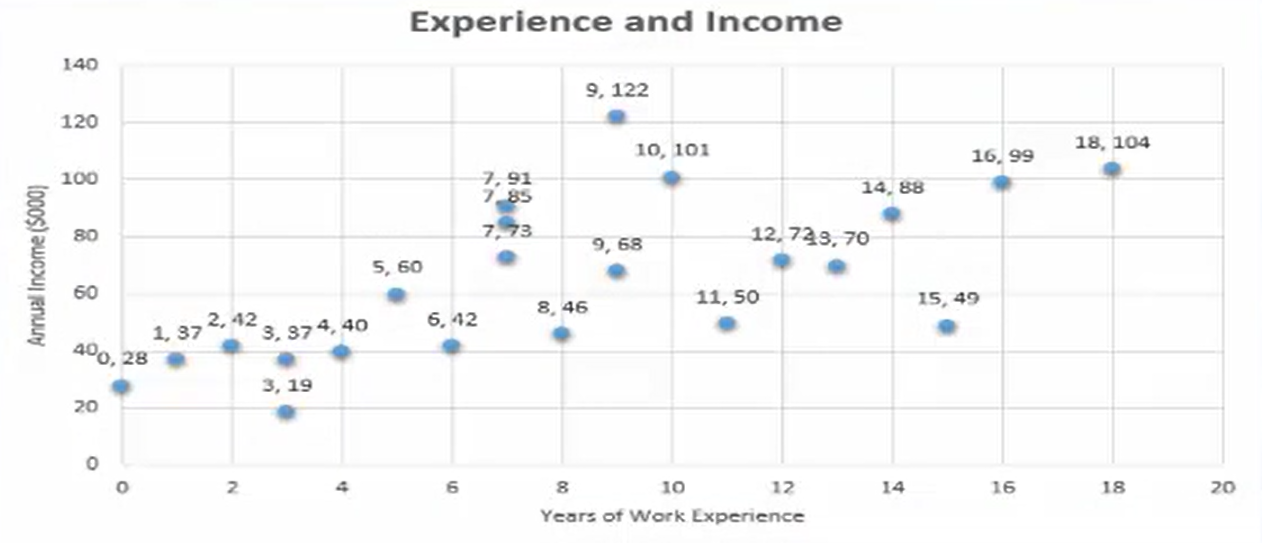There are variables that are related in every aspect of nature, such as the amount of exercise a person engages in and their resting heart rate. In the event that two variables are related, they are said to be correlated.

The two simplest ways that two variables can be correlated are if one variable increases and the other increases or if one variable increases, the other decreases.

If the second variable increases when the first one increases, the scatterplot shows an upward trend, meaning that the points in the scatterplot increase from left to right. An upward trend like this is referred to as a positive association between variables.

We would intuitively expect a positive association between a person’s education level and his or her income. As you can see, based upon the scatterplot and the trend line on this graph in front of you, it is.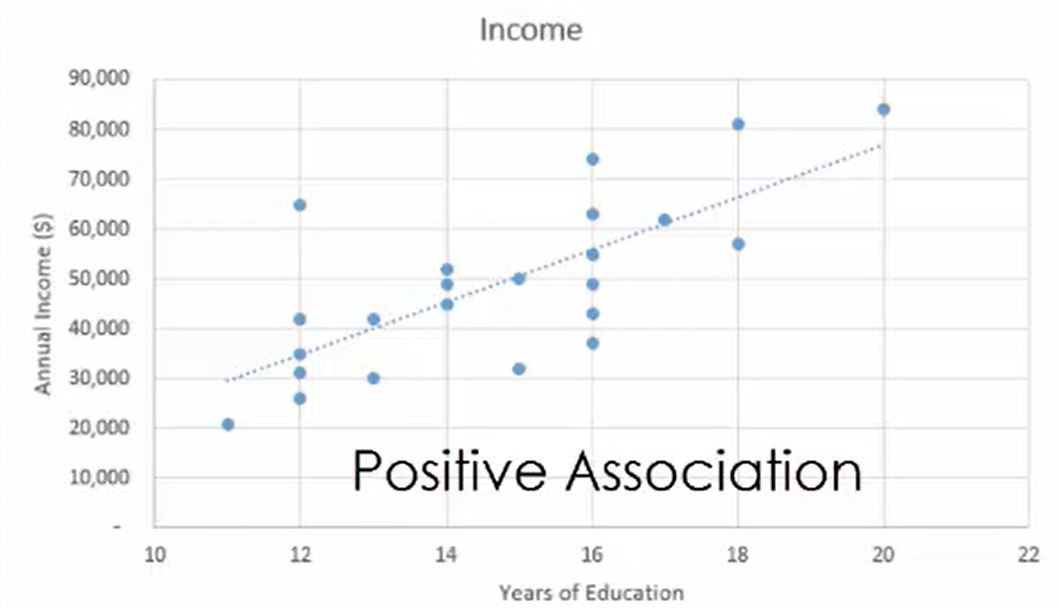If the second variable decreases as the first one increases, this can be seen in the scatterplot as a downward trend where the points of the scatterplot fall from left to right. This downward trend is called a negative association between the variables.

The number of absences a student has and his or her grade point average have just such a negative relationship. The less often a student is in class or the more absences he or she has, the more likely his or her grades are to suffer.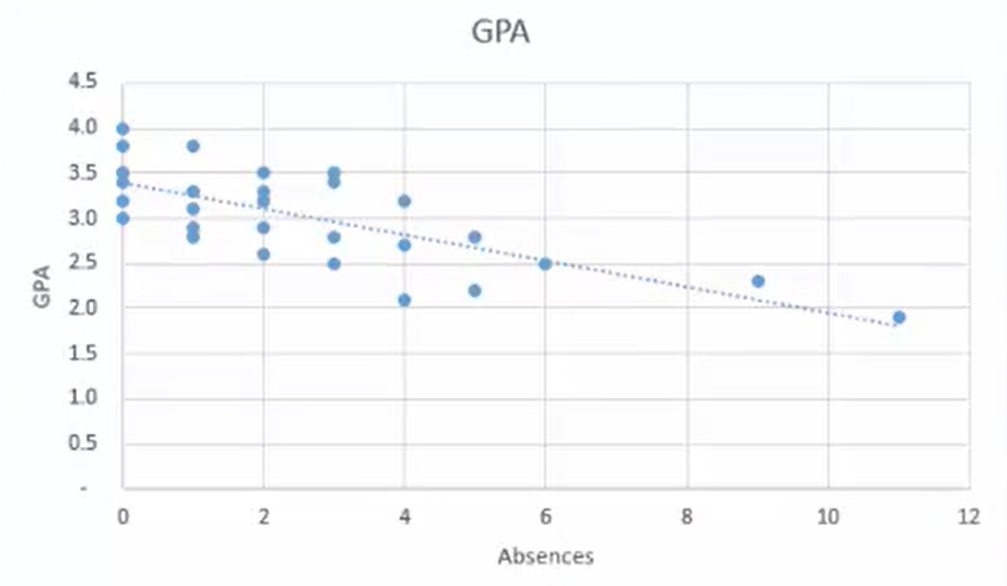It is important to identify trends in data, because they can help establish a relationship between variables, especially when looking at them in a scatterplot. If you were to look at a scatterplot that showed a person’s age and his or her annual healthcare expenditures, you would likely get a sense that variables such as these have a positive association. This is because older people would typically have more health issues. As a result, they will probably have greater healthcare expenditures.

By recognizing trends in data, we might also be able to better predict what could happen in a specific scenario. If an independent variable increases and you know that the independent variables are associated negatively, you could predict that the dependent variable would decrease as well.

Suppose you compared the heights of 40 girls relative to their ages. Intuitively you would expect that to be a positive association.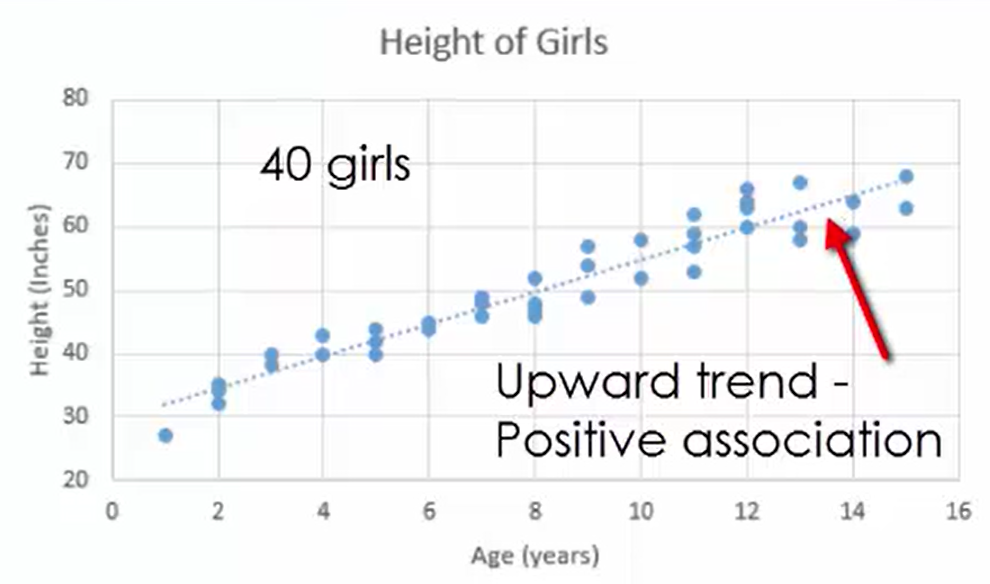There’s an upward trend. Each data point you see here represents two different values: how old each girl is in years and her height in inches. Notice that the upper trend here follows this particular line. It’s a relatively strong trend.

Now let’s look at something that might have a negative association, such as the number of hours of television a student watches per week relative to that student’s grade point average. Notice that there’s a downward trend, as you might expect.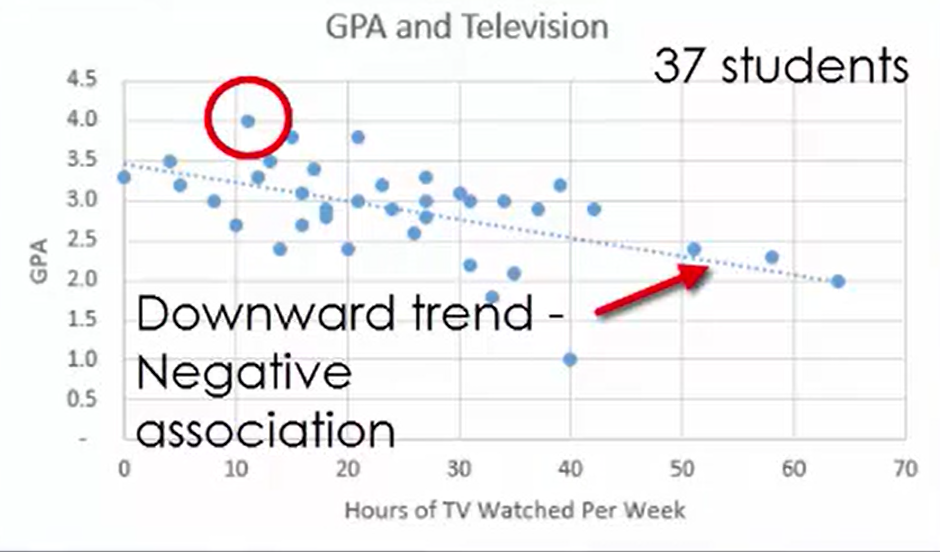Each data point here represents two measurements: the hours of television the student watches and the student’s grade point average. You can see that this means that the more television a student watches, probably the less time he or she is studying. As a result, grades tend to go down, and it tends to follow the line you see illustrated here.

## 2. Correlation Coefficients

A numerical value called a correlation coefficient is used to indicate an upward or downward trend in a scatterplot. A correlation coefficient also indicates how well the data on a scatterplot follows a straight line. The correlation coefficient is denoted by the symbol r and is a number that always lies between -1 and 1. If the correlation coefficient is 0, there is no upward or downward trend in the scatterplot. The line is either a flat horizontal line or the data may be so scattered that it does not follow any noticeable pattern.

When the correlation coefficient is positive, there is an upward trend in the scatterplot. When the correlation coefficient is negative, there is a downward trend in the scatterplot. If the correlation coefficient is positive and near 1, an upward trend exists in the scatterplot that follows a straight line. If the correlation coefficient is negative and near -1, there exists a downward trend in the scatterplot, and that would closely follow a straight line as well.

The sign of the correlation coefficient, whether it is positive or negative, illustrates the direction of the trend or association between the two variables. The proximity of this correlation coefficient to 1 or negative 1 reveals the strength of the trend or association between these two variables. When the correlation coefficient is positive and close to 1, there is a strong positive association between the two variables.

When the correlation coefficient is negative and nearer to -1, there is a strong negative association between the variables. If the correlation coefficient happens to be positive but closer to 0, there is a weak positive association between the variables. If the correlation coefficient is negative but closer to 0, there would be a weak negative association between the two variables.

• Positive, close to 1: Strong Positive Association.
• Postitive, close to 0: Weak Positive Association.
• Negative, close to -1: Strong Negative Association.
• Negative, close to 0: Weak Negative Association.
term to know

Correlation Coefficient (r)
A number that indicates an upward or downward trend in the scatterplot, and also how well the trend follows a straight line

## 3. Correlation Coefficients on Scatterplots

Here is a scatterplot illustrating the relationship between a person’s income and how much money he or she has saved for retirement. Notice that you’ll see the particular value listed here as the correlation coefficient.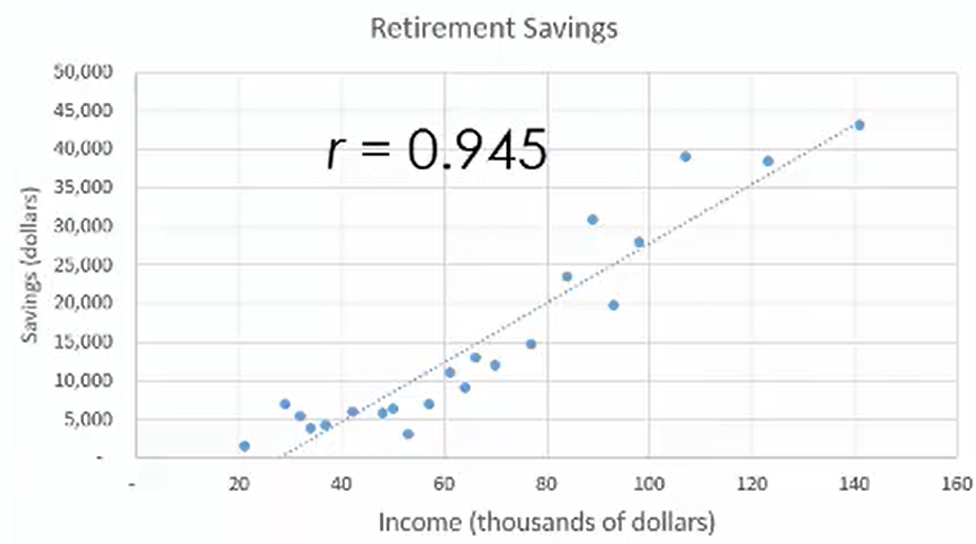The correlation coefficient is equal to a 0.945, which is very strong, and it’s positive. The more money somebody earns, in all likelihood, the more he or she is able to save for retirement. There’s a very, very strong association between those two variables.

This scatterplot illustrates something completely different. It’s dealing with the number of hours per month that a golfer practices his or her swing relative to his or her average score.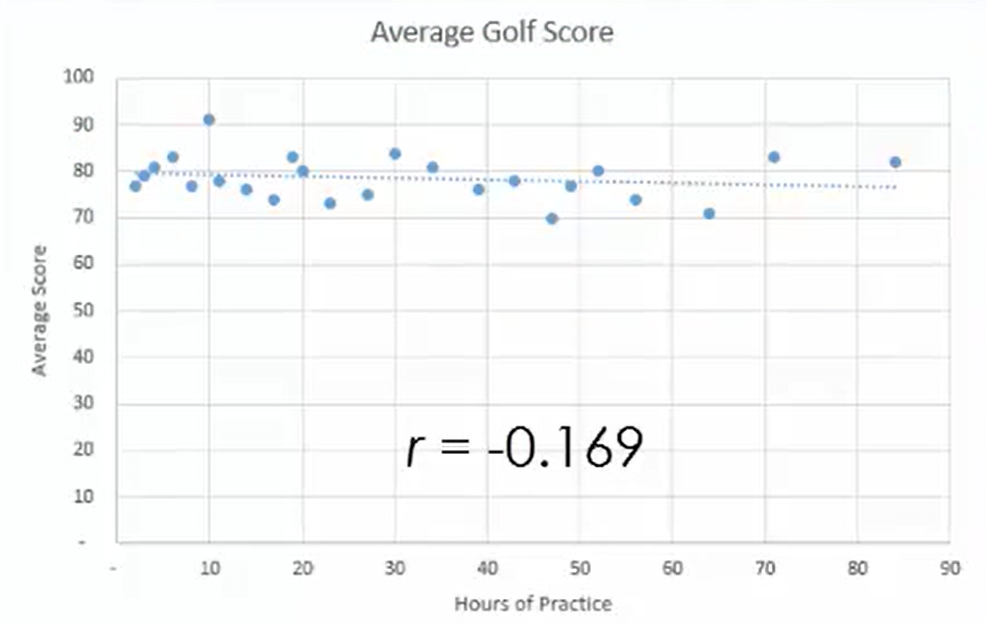As you see with the scatterplot, there is not much of a downward association here. The correlation coefficient is equal to -0.169.

What does this this tell you?

You would expect that the more somebody practices, the better his or her golf score would be. A better golf score would be a lower score. However, it’s not a very strong association.

Now look at the weight of cars and the miles per gallon that they get. You would expect this one intuitively to be a very strong negative association.

What does this mean?

This means that the variables of weight and gas mileage would be closely related.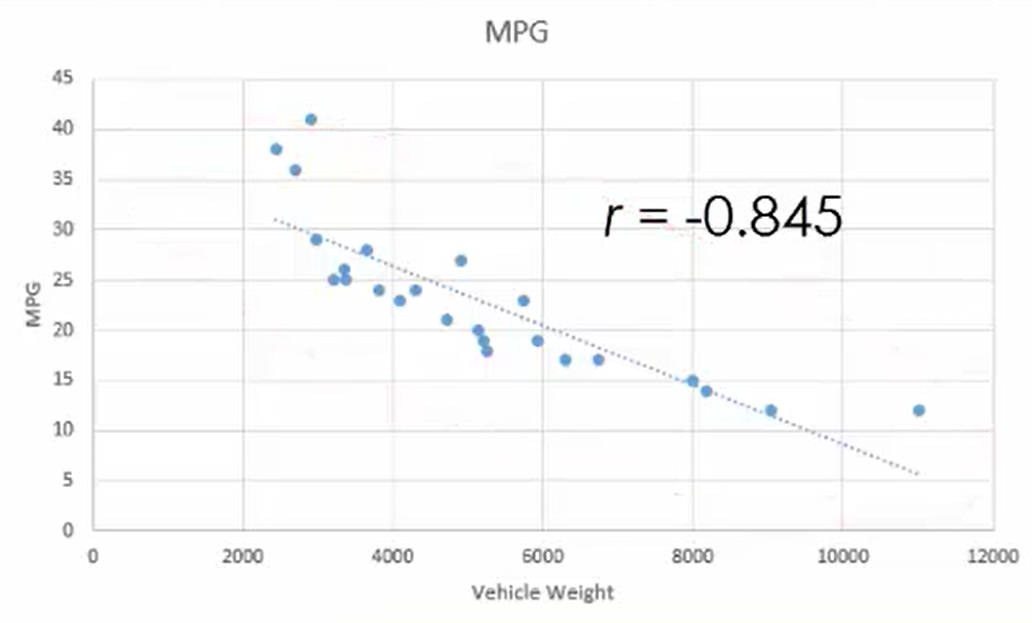A heavier vehicle would probably get worse gas mileage, which would be a lower miles-per-gallon figure. As you see by the scatterplot, there’s a downward-sloping line. The correlation coefficient is -0.845. Since that value is close to negative 1, you can tell that it’s a negative association that is relatively strong.

Take a look at how much somebody weighs relative to how much ice cream he or she eats in a month. Say you ask a group of people how many ice cream cones they consume in a month and what their weight is. Maybe you would expect there to be a positive correlation here, meaning that if you eat a high-calorie foods like ice cream, you might be more prone to gain weight.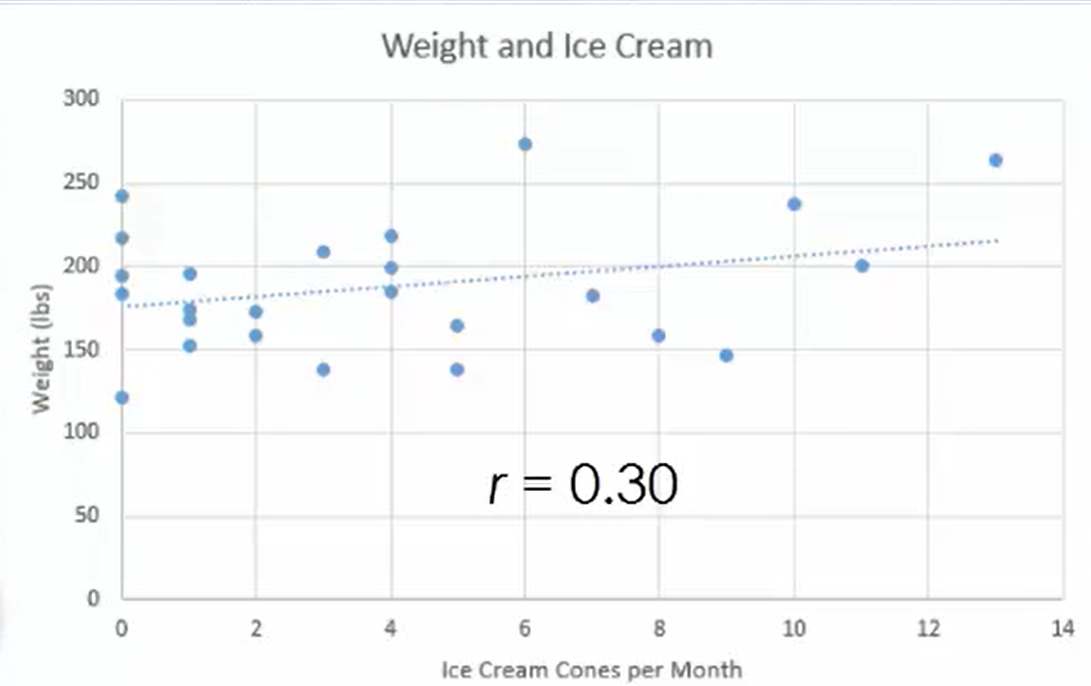As you see from the scatterplot, there is a positive association, but it’s a very weak one. The correlation coefficient is 0.30, which tells us it’s relatively weak simply because it’s closer to 0 than it would be to 1.

summary
Scatterplots are used to show a cause-and-effect relationship between two variables. They are for interval or ratio variables. If two variables are related, they are said to have a correlation. The correlation coefficient is a number between -1 and 1 that describes how strong a relationship is and whether is it positive or negative. You learned about correlation coefficients on scatterplots to see how this works.

Source: This work is adapted from Sophia author Dan Laub.

Terms to Know
Correlation Coefficient (r)

A number that indicates whether there is an upward trend or a downward trend in the scatterplot, and also how well the trend follows a straight line.

Rating